# The Quadratic Programming Solver

### Example 9.3 Portfolio Selection with Transactions

Consider a portfolio selection problem with a slight modification. You are now required to take into account the current position and transaction costs associated with buying and selling assets. The objective is to find the minimum variance portfolio. In order to understand the scenario better, consider the following data.

You are given three assets. The current holding of the three assets is denoted by the vector= [200, 300, 500], the amount of asset bought and sold is denoted by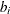and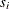, respectively, and the net investment in each asset is denoted byand is defined by the following relation: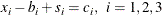Suppose that you pay a transaction fee of 0.01 every time you buy or sell. Let the covariance matrix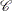be defined as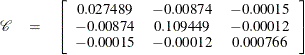Assume that you hope to obtain at least 12% growth. Let= [1.109048, 1.169048, 1.074286] be the vector of expected return on the three assets, and let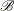=1000 be the available funds. Mathematically, this problem can be written in the following manner: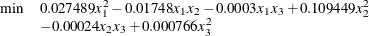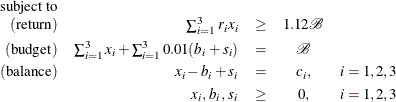The problem can be solved by the following SAS statements:

/* example 3: portfolio selection with transactions */
proc optmodel;
/* let x1, x2, x3 be the amount invested in each asset */
var x{1..3} >= 0;
/* let b1, b2, b3 be the amount of asset bought */
var b{1..3} >= 0;
/* let s1, s2, s3 be the amount of asset sold */
var s{1..3} >= 0;

/* current holdings */
num c{1..3}=[ 200 300 500];
/* covariance matrix */
num coeff{1..3, 1..3} = [0.027489  -.008740  -.000150
-.008740  0.109449  -.000120
-.000150  -.000120  0.000766];
/* returns */
num r{1..3}=[1.109048 1.169048 1.074286];

/* minimize the variance of the portfolio's total return */
minimize f = sum{i in 1..3, j in 1..3}coeff[i,j]*x[i]*x[j];

/* subject to the following constraints */
con BUDGET: sum{i in 1..3}(x[i]+.01*b[i]+.01*s[i]) <= 1000;
con RETURN: sum{i in 1..3}r[i]*x[i] >= 1120;
con BALANC{i in 1..3}: x[i]-b[i]+s[i]=c[i];

solve with qp;

/* print the optimal solution */
print x;
quit;


The output is displayed in Output 9.3.1.

Output 9.3.1: Portfolio Selection with Transactions

The OPTMODEL Procedure

Problem Summary
Objective Sense Minimization
Objective Function f

Number of Variables 9
Bounded Above 0
Bounded Below 9
Bounded Below and Above 0
Free 0
Fixed 0

Number of Constraints 5
Linear LE (<=) 1
Linear EQ (=) 3
Linear GE (>=) 1
Linear Range 0

Constraint Coefficients 21

Performance Information
Execution Mode Single-Machine
Number of Threads 4

Solution Summary
Solver QP
Algorithm Interior Point
Objective Function f
Solution Status Optimal
Objective Value 19560.725753

Primal Infeasibility 1.103614E-16
Dual Infeasibility 2.559425E-14
Bound Infeasibility 0
Duality Gap 7.221601E-16
Complementarity 0

Iterations 11
Presolve Time 0.00
Solution Time 0.02

 x
1 397.58
2 406.12
3 190.17